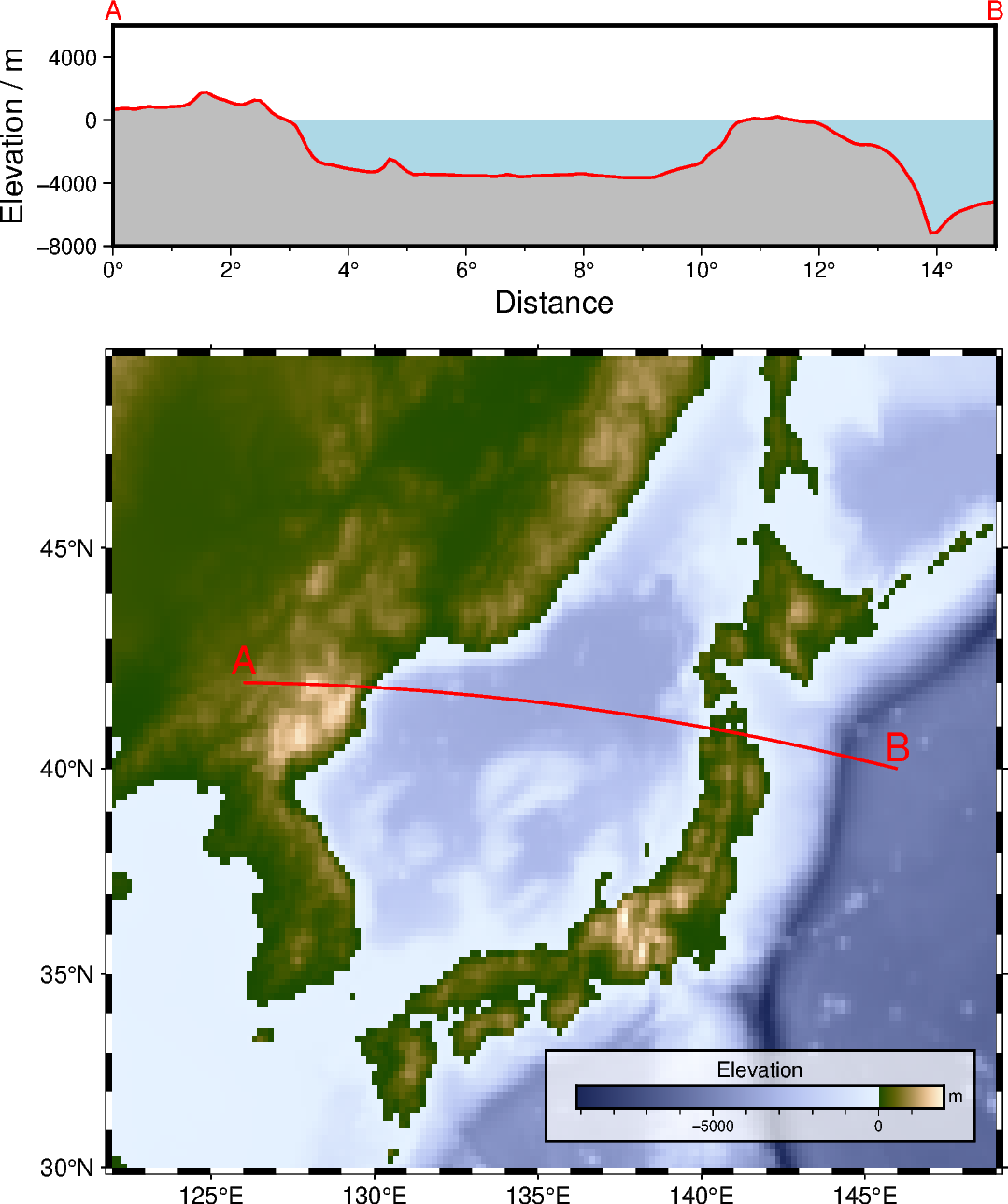# Cross-section along a transect

`pygmt.project` and `pygmt.grdtrack` can be used to focus on a quantity and its variation along a desired survey line. In this example, the elevation is extracted from a grid provided via `pygmt.datasets.load_earth_relief`. The figure consists of two parts, a map of the elevation in the study area showing the survey line and a Cartesian plot showing the elevation along the survey line.

This example is orientated on an example in the GMT/China documentation: https://docs.gmt-china.org/latest/examples/ex026/```import pygmt

# Define region of study area
# lon_min, lon_max, lat_min, lat_max in degrees East and North
region_map = [122, 149, 30, 49]

# Create a new pygmt.Figure instance
fig = pygmt.Figure()

# ----------------------------------------------------------------------------
# Bottom: Map of elevation in study area

# Set up basic map
fig.basemap(
region=region_map,
projection="M12c",  # Mercator projection with a width of 12 centimeters
frame="af",
)

# Download grid for Earth relief with a resolution of 10 arc-minutes and
# gridline registration [Default]
resolution="10m",
region=region_map,
)

fig.grdimage(grid=grid_map, cmap="oleron")

# Add a colorbar for the elevation
fig.colorbar(
# Place the colorbar inside the plot (lower-case "j") with justification
# Bottom Right and an offset ("+o") of 0.7 centimeters and
# 0.3 centimeters in x or y directions, respectively
# Move the x label above the horizontal colorbar ("+ml")
position="jBR+o0.7c/0.8c+h+w5c/0.3c+ml",
# Add a box around the colobar with a fill ("+g") in "white" color and
# a transparency ("@") of 30 % and with a 0.8-points thick black
# outline ("+p")
box="+gwhite@30+p0.8p,black",
# Add x and y labels ("+l")
frame=["x+lElevation", "y+lm"],
)

# Choose a survey line
fig.plot(
x=[126, 146],  # Longitude in degrees East
y=[42, 40],  # Latitude in degrees North
# Draw a 2-points thick red dashed line for the survey line
pen="2p,red,dashed",
)

# Add labels "A" and "B" for the start and end points of the survey line
fig.text(
x=[126, 146],
y=[42, 40],
text=["A", "B"],
offset="0c/0.2c",  # Move text 0.2 centimeters up (y direction)
font="15p",  # Use a font size of 15 points
)

# ----------------------------------------------------------------------------
# Top: Elevation along survey line

# Shift plot origin 12.5 centimeters to the top
fig.shift_origin(yshift="12.5c")

fig.basemap(
region=[0, 15, -8000, 6000],  # x_min, x_max, y_min, y_max
# Cartesian projection with a width of 12 centimeters and
# a height of 3 centimeters
projection="X12c/3c",
# Add annotations ("a") and ticks ("f") as well as labels ("+l")
# at the west or left and south or bottom sides ("WSrt")
frame=["WSrt", "xa2f1+lDistance+u°", "ya4000+lElevation / m"],
)

# Add labels "A" and "B" for the start and end points of the survey line
fig.text(
x=[0, 15],
y=[7000, 7000],
text=["A", "B"],
no_clip=True,  # Do not clip text that fall outside the plot bounds
font="10p",  # Use a font size of 10 points
)

# Generate points along a great circle corresponding to the survey line
# and store them in a pandas.DataFrame
track_df = pygmt.project(
center="126/42",  # Start point of survey line (longitude/latitude)
endpoint="146/40",  # End point of survey line (longitude/latitude)
generate="0.1",  # Output data in steps of 0.1 degrees
)

# Extract the elevation at the generated points from the downloaded grid
# and add it as new column "elevation" to the pandas.DataFrame
track_df = pygmt.grdtrack(
grid=grid_map,
points=track_df,
newcolname="elevation",
)

# Plot water masses
fig.plot(
x=[0, 15],
y=[0, 0],
fill="lightblue",  # Fill the polygon in "lightblue"
# Draw a 0.25-points thick black solid outline
pen="0.25p,black,solid",
close="+y-8000",  # Force closed polygon
)

# Plot elevation along the survey line
fig.plot(
x=track_df.p,
y=track_df.elevation,
fill="gray",  # Fill the polygon in "gray"
# Draw a 1-point thick black solid outline
pen="1p,black,solid",
close="+y-8000",  # Force closed polygon
)

fig.show()
```

Total running time of the script: ( 0 minutes 1.774 seconds)

Gallery generated by Sphinx-Gallery